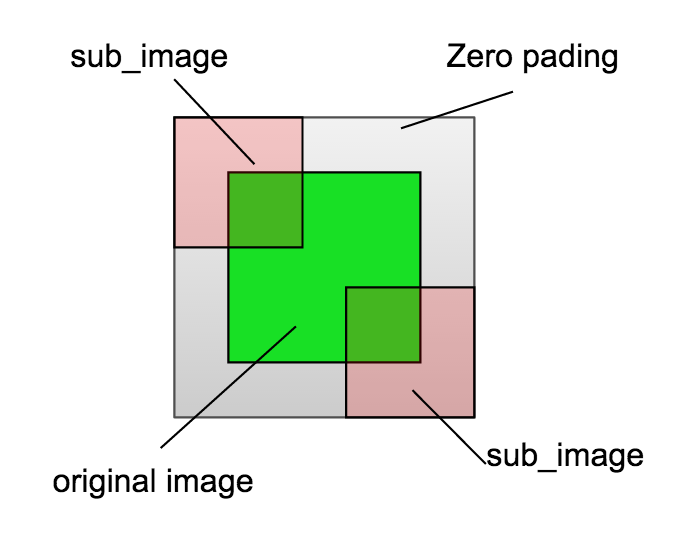The post records some notes for CNN Face Detection project in my PhD in the University of Nottingham.

### Note 1: Make image square and crop/split it into sub_images

Make image square

In order to use Convolutional Neural Network that (mostly) requires the input image square, i.e. of shape (3, N, N), I need to make the height equals to width. There are 3 ways coming into my mind:

• Stretch the image to square, not good because the face could be stretched.
• Crop the image to square, usually set the cropped size as the smaller one of width and height. Not good because information could be lost
• Padded the image with zeros to square, better solution. However we need to store the padded size in order to convert from padded image coordinates to original image coordinates. We can define a new class SuperImage to achieve this.

Split image into sub_images

For simplicity (because simple is good), I use sliding window to split images. I design to overlap the sub_images to make sure any two continuous pixels can appear at least one sub_image. Specifically, if I want to get n*n sub_images, then set stride = int(1.0/n * height), and sub_image size sub_size = 2 * stride to overlap half of the sub_images. If the size of remains are not enough to form a sub_image, we can add zero_padding or just throw them away. In addition, we can use SubImage Class to store the information to convert sub_image coordinates back to original image.

Convert from subimage to original image

The process is a little tricky. Because the subimages are actually cropped from padded image, the coordinates of subimages in the coordinate of original image can be less than 0, and also bigger than the original image size (see following figure). So we should make sure the details right when convert subimage information (e.g. human face bounding box) to original image coordinates.Following code illustrate how to convert a subimage heatmap to the corresponding position in the coordinates of the original image